0%

## A Comprehensive Survey on Graph Neural Networks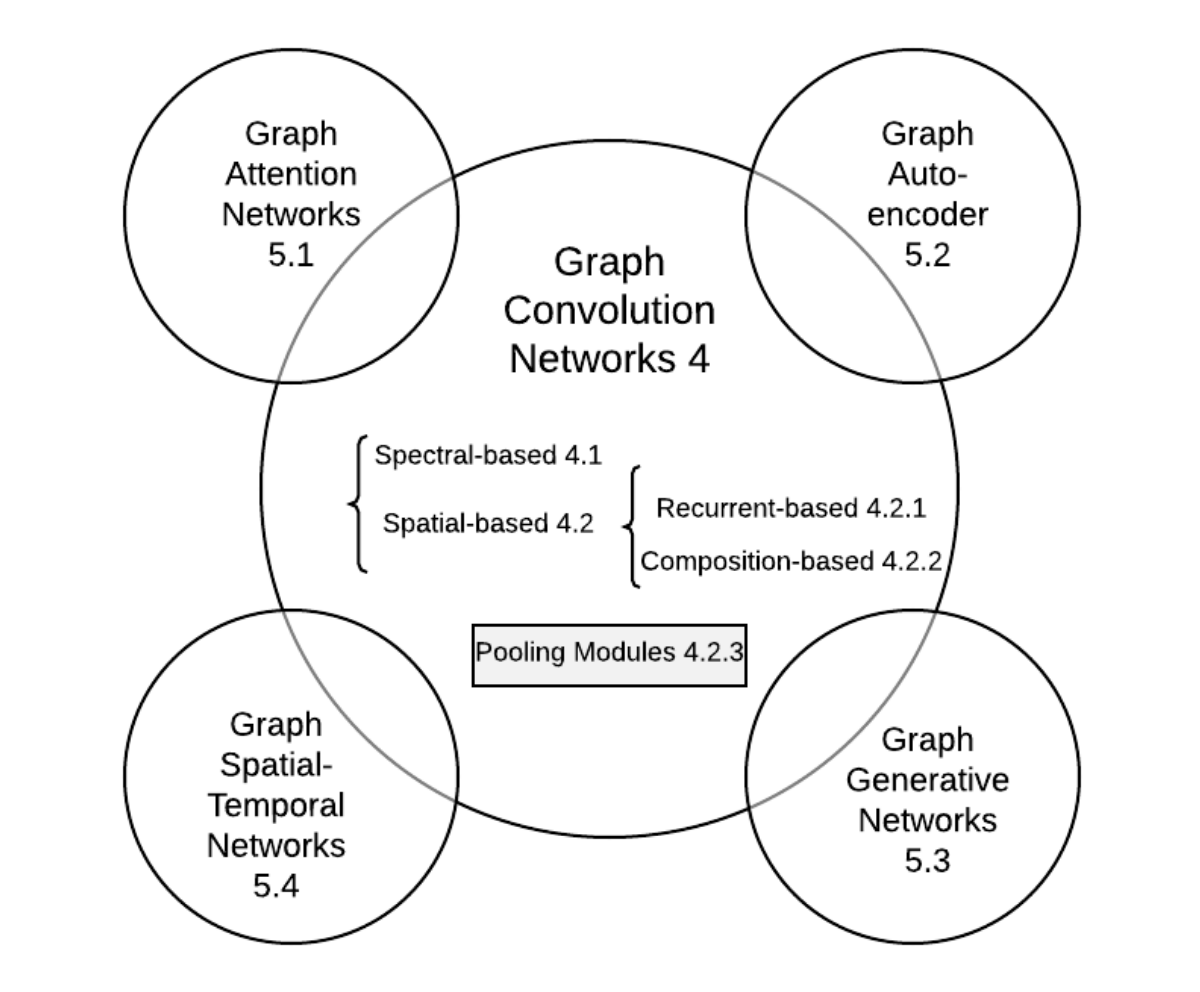• GNN分类（按更新方式）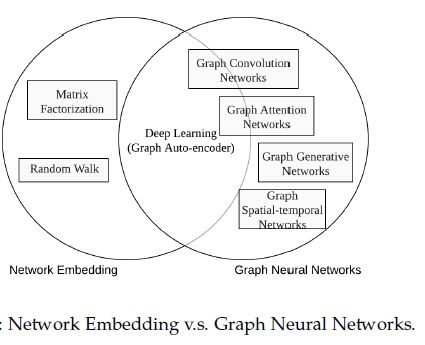• 与图嵌入的关系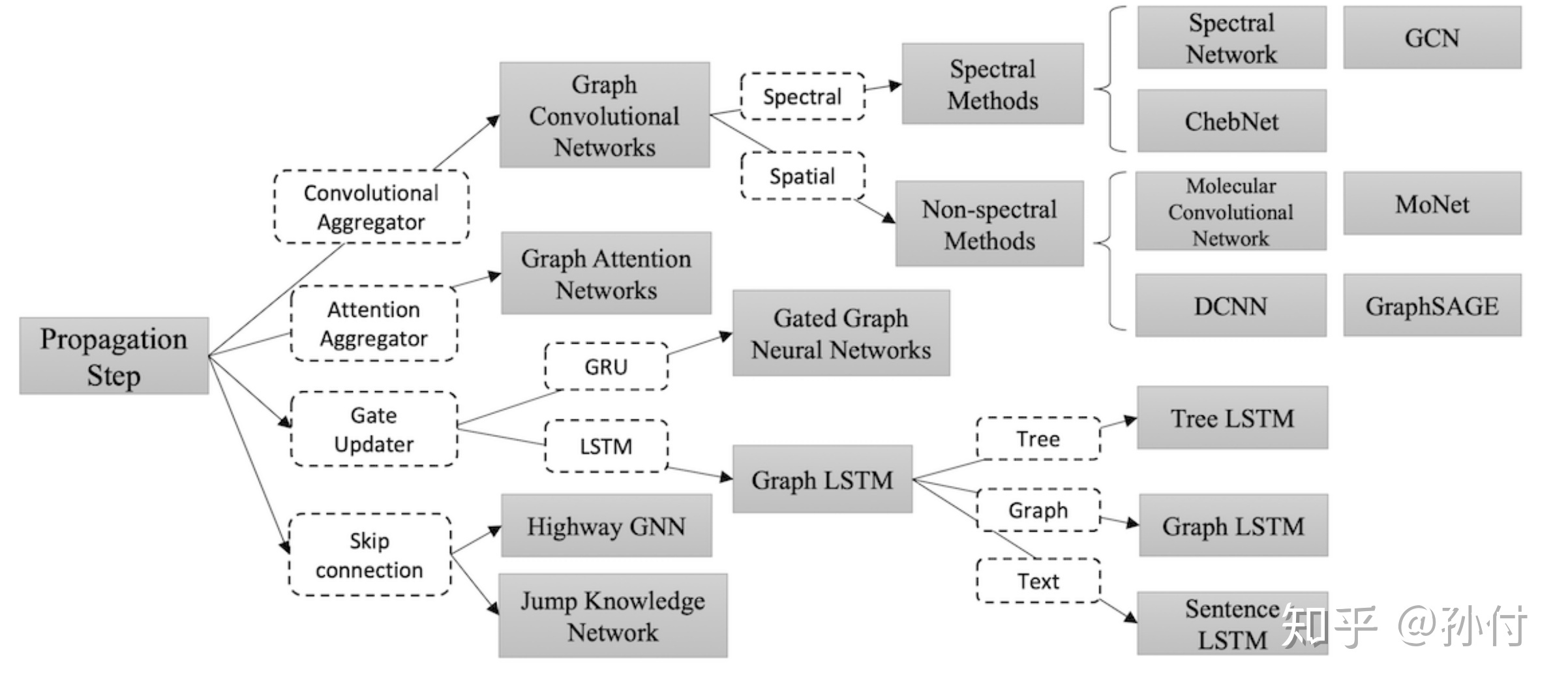## Revisiting Semi-Supervised Learning with Graph Embeddings

#### Graph-Based Semi-Supervised Learning

• Graph-based semi-supervised learning is based on the assumption that nearby nodes tend to have the same labels.

Loss:

• the first term is the standard supervised loss function
• The second term is the graph Laplacian regularization, which incurs a large penalty when similar nodes with a large $w_{ij}$ are predicted to have different labels

### Learning Embeddings

A number of embedding learning methods are based on the Skipgram model, which is a variant of the softmax model

Loss:

• $C$ is the set of all possible context
• $w$ are parameters of the Skipgram model
• $e_i$ is the embedding of instance $i$

Application:

• word2vec(NLP)
• Deepwalk(Graph)
• LINE(multiple context spaces)

### Semi-Supervised Learning with Graph Embeddings

Loss:

• $\mathcal{L}_s$ is a supervised loss of predicting the labels
• $\mathcal{L}_u$ is an unsupervised loss of predicting the graph context

#### Sampling Context

We formulate the unsupervised loss $\mathcal{L}_u$ as a variant of Skipgram loss

negative sampling:

we are sampling $(i, c, γ)$ from a distribution, where $i$ and $c$ denote instance and context respectively, $γ = +1$ means $(i, c)$ is a positive pair and $γ = −1$ means negative. Given $(i, c, γ)$, we minimize the cross entropy loss of classifying the pair $(i, c)$ to a binary label $γ$:

sampling algorithm: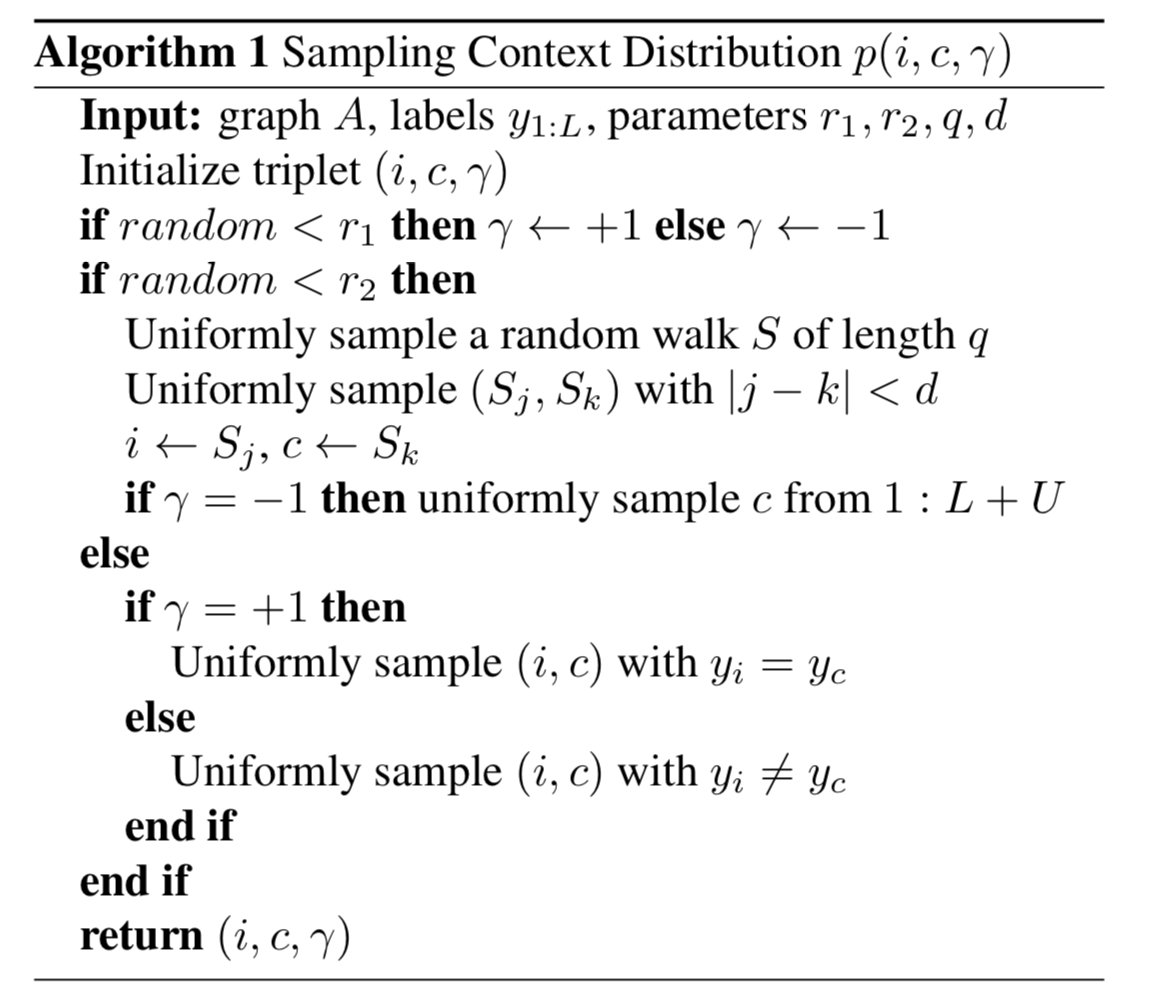example: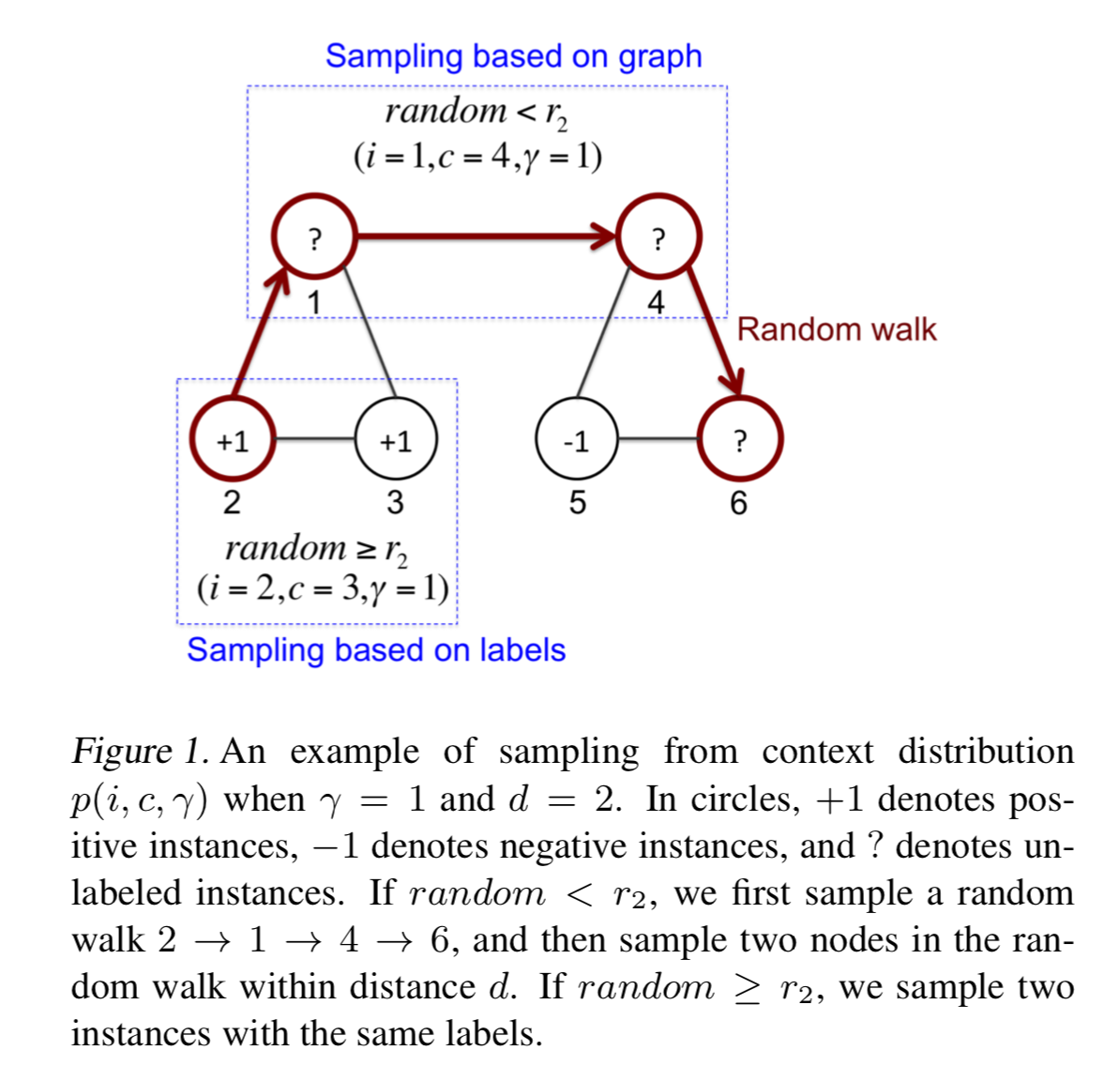#### Transductive Formulation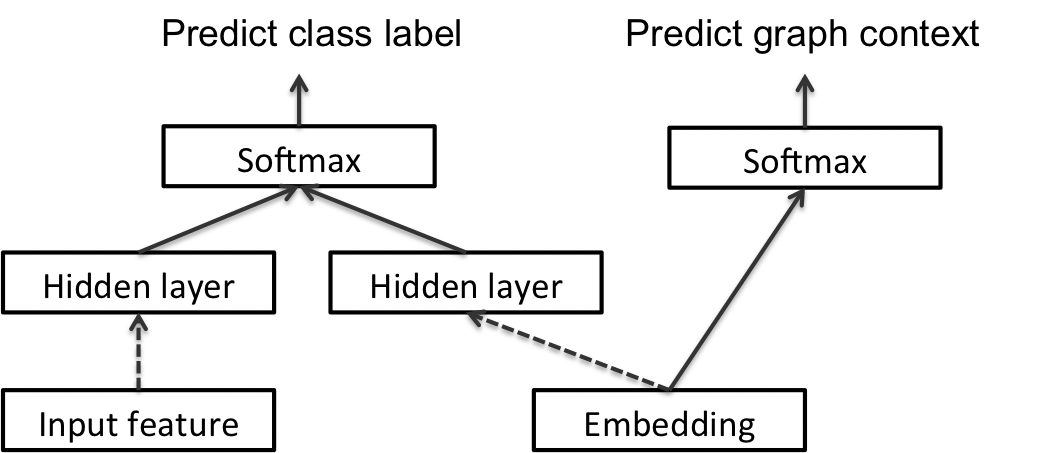The two hidden layers are concatenated, and fed to a softmax layer to predict the class label of the instance

#### Inductive Formulation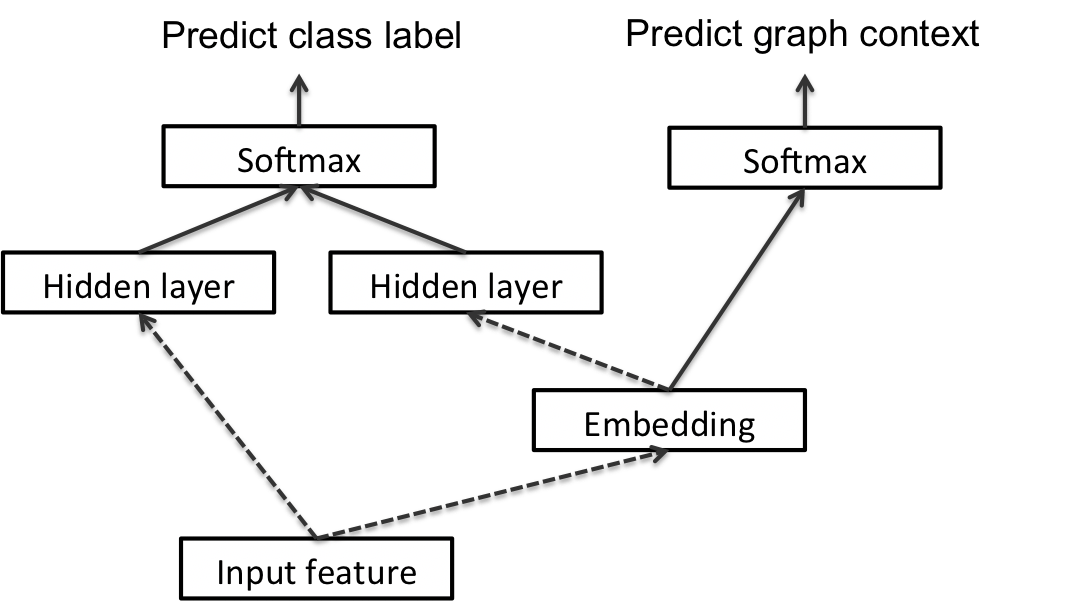### Conclusion

contributions:

• incontrast to previous semi-supervised learning approaches that largely depend on graph Laplacian regularization, we propose a novel approach by joint training of classification and graph context prediction
• since it is difficult to generalize graph embeddings to novel instances, we design a novel inductive approach that conditions embeddings on input features

## ANRL: Attributed Network Representation Learning via Deep Neural Networks

issues：

• network topology and node attributes are two heterogeneous information sources, thus it is challenging to preserve their properties in a common vector space
• the observed network data is often incomplete and even noisy, which brings more difficulties for obtaining informative representations

contributions:

• integrates network structural proximity and node attributes affinity into low-dimensional representation spaces
• we design a neighbor enhancement autoencoder, which can retain better similarity between data samples in the representation space
• We also propose an attribute-aware skip-gram model to capture the structure correlations
• These two components share connections to the encoder

### Proposed Model

#### problem formulation

we aim to represent:

• each node $i ∈ V$ as a low-dimensional vector $y_i$
• mapping function f preserves not only network structure but also node attribute proximity

#### neighbor enhancement autoencoder

Loss:

• $\hat{x}_i$ is the reconstruction output of decoder
• $T(v_i)$ returns the target neighbors of $v_i$
• $T(·)$ incorporates prior knowledge into the model and can be adopted by the following two formulations:
• Weighted Average Neighbor
• Elementwise Median Neighbor

#### attribute-aware skip-gram model

Loss:

where:

• $v_{i+j}$ is the node context in the generated random sequence
• $b$ is the window size

negative sampling:

for a specific node-context pair $(v_i,v_{i+j})$, we have the following objective:

where:

• $P_n(v) ∝ d_v^{3/4}$ as suggested in [Mikolov et al., 2013b]
• where $d_v$ is the degree of node v

#### joint optimization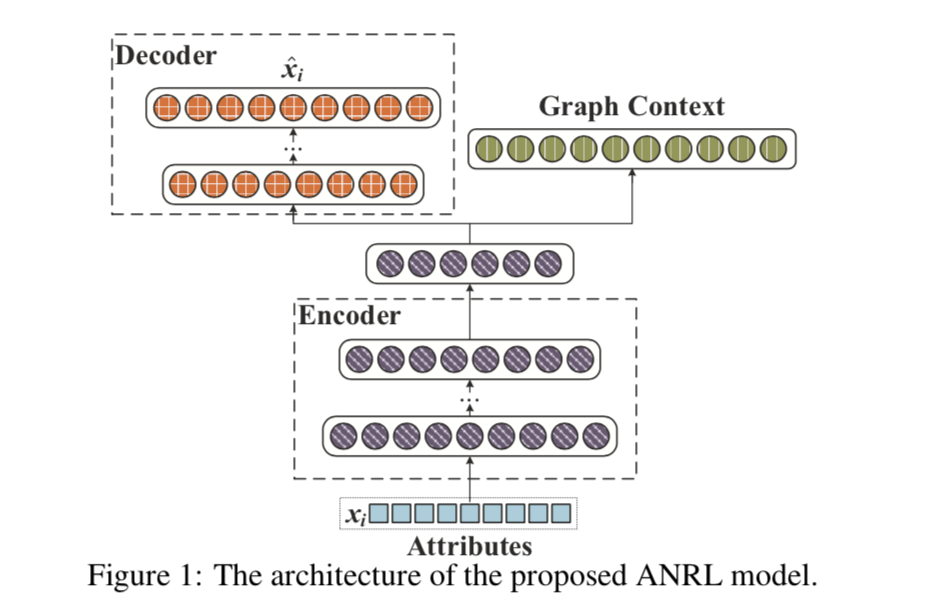Loss:

### Conclusions

• incorporates both node attributes and network structure information into the embedding
• To further address the structure proximity and attribute affinity preserving, we develop a neighbor enhancement autoencoder and attribute-aware skip-gram model to exploit the complex interrelations between structural information and attributes

## DeepWalk: Online Learning of Social Representations

the sparsity of a network representation is both a strength and a weakness:

• Sparsity enables the design of efficient discrete algorithms
• but can make it harder to generalize in statistical learning

We develop an algorithm (DeepWalk) that learns social representations of a graph’s vertices, by modeling a stream of short random walks

DeepWalk takes a graph as input and produces a latent representation as an output

We propose an unsupervised method which learns features that capture the graph structure independent of the labels’ distribution.

### Learning Social Representations

We seek learning social representations with the following characteristics:

• Adaptability - Real social networks are constantly evolving; new social relations should not require repeating the learning process all over again.
• Community aware - The distance between latent dimensions should represent a metric for evaluating social similarity between the corresponding members of the network. This allows generalization in networks with homophily.
• Low dimensional - When labeled data is scarce, low-dimensional models generalize better, and speed up convergence and inference.
• Continuous - We require latent representations to model partial community membership in continuous space. In addition to providing a nuanced view of community membership, a continuous representation has smooth decision boundaries between communities which allows more robust classification.

#### random walks

We denote a random walk rooted at vertex $v_i$ as $W_{v_i}$ . It is a stochastic process with random variables $W_{v_i}^1 ,W_{v_i}^2 ,…,W_{v_i}^k$
such that $W_{v_i}^{k + 1}$ is a vertex chosen at random from the neighbors of vertex $v_k$

#### connection: power laws

we observe that the frequency which vertices appear in the short random walks will also follow a power-law distribution
word frequency in natural language follows a similar distribution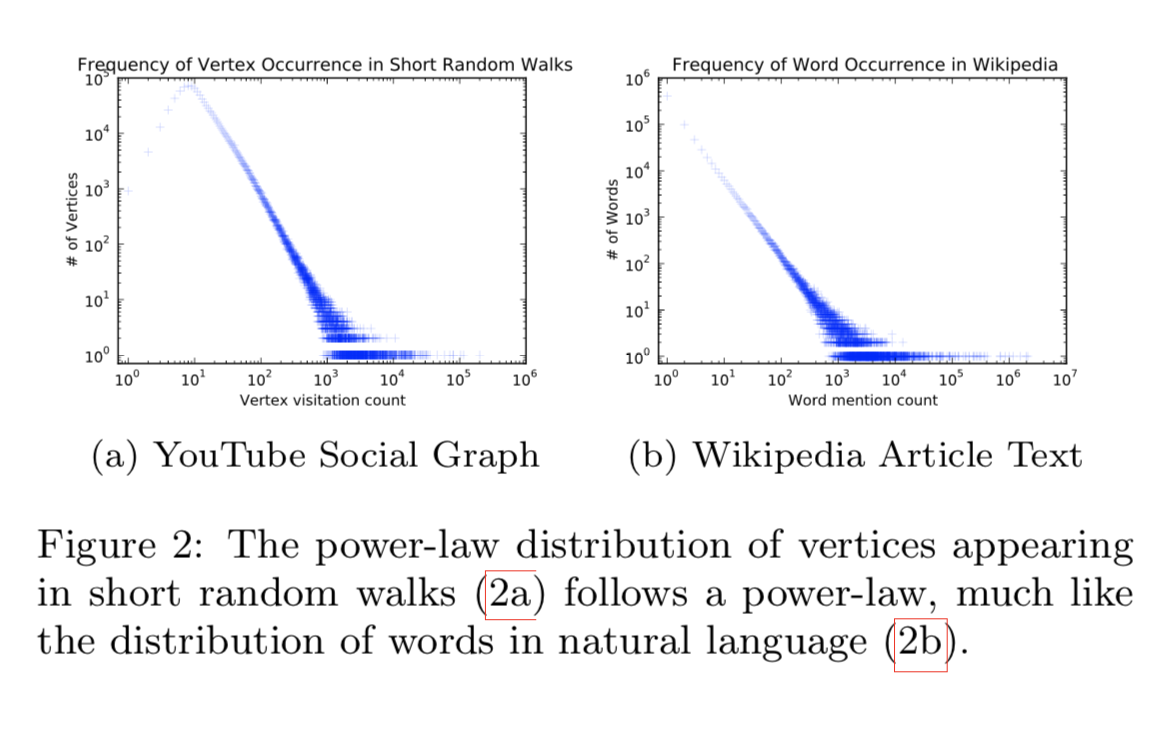#### Language Modeling

relaxations：

from

to

• First, the order independence assumption better captures a sense of nearness that is provided by random walks.
• Moreover, this relaxation is quite useful for speeding up the training time by building small
models as one vertex is given at a time

### Method

#### DeepWalk

the algorithm consists of two main components:

• a random walk generator
• an update procedure

A walk samples uniformly from the neighbors of the last vertex visited until the maximum $length(t)$ is reached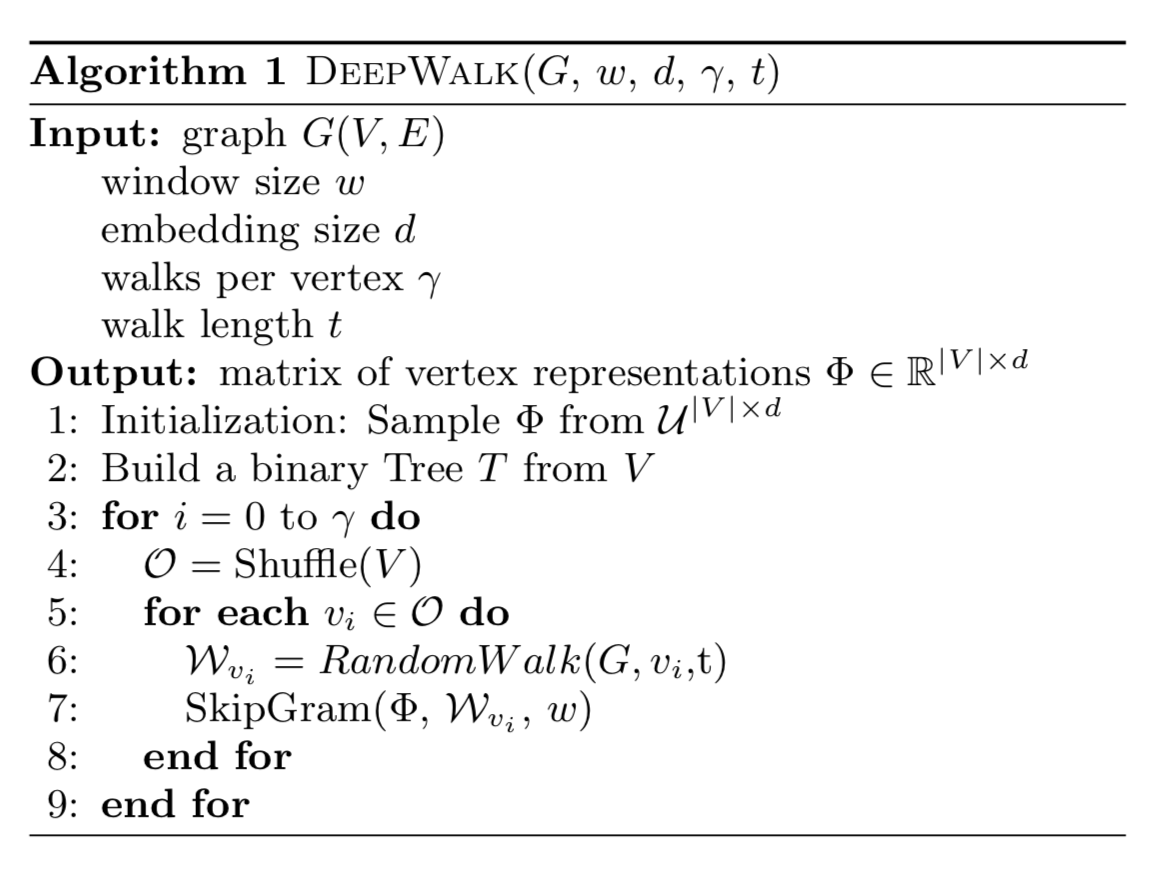At the start of each pass we generate a random ordering to traverse the vertices. This is not strictly required, but is well-known to speed up the convergence of stochastic gradient descent.

##### skipgram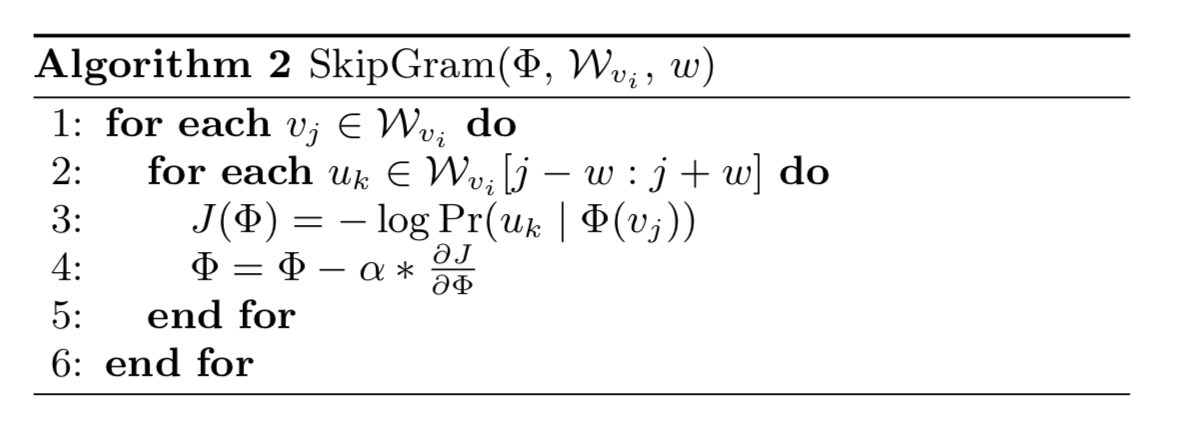To speed the training time, Hierarchical Softmax can be used to approximate the probability distribution

##### hierarchical softmax

we assign the vertices to the leaves of a binary tree, the prediction problem turns into maximizing the probability of a specific path in the tree

Huffman coding is used to reduce the access time of frequent elements in the tree

### Variants

• Streaming
• Non-random walks

## LINE: Large-scale Information Network Embedding

• We propose a novel network embedding model called the “LINE,” which suits arbitrary types of information networks and easily scales to millions of nodes. It has a carefully designed objective function that preserves both the first-order and second-order proximities.
• We propose an edge-sampling algorithm for optimizing the objective. The algorithm tackles the limitation of the classical stochastic gradient decent and improves the effectiveness and efficiency of the inference.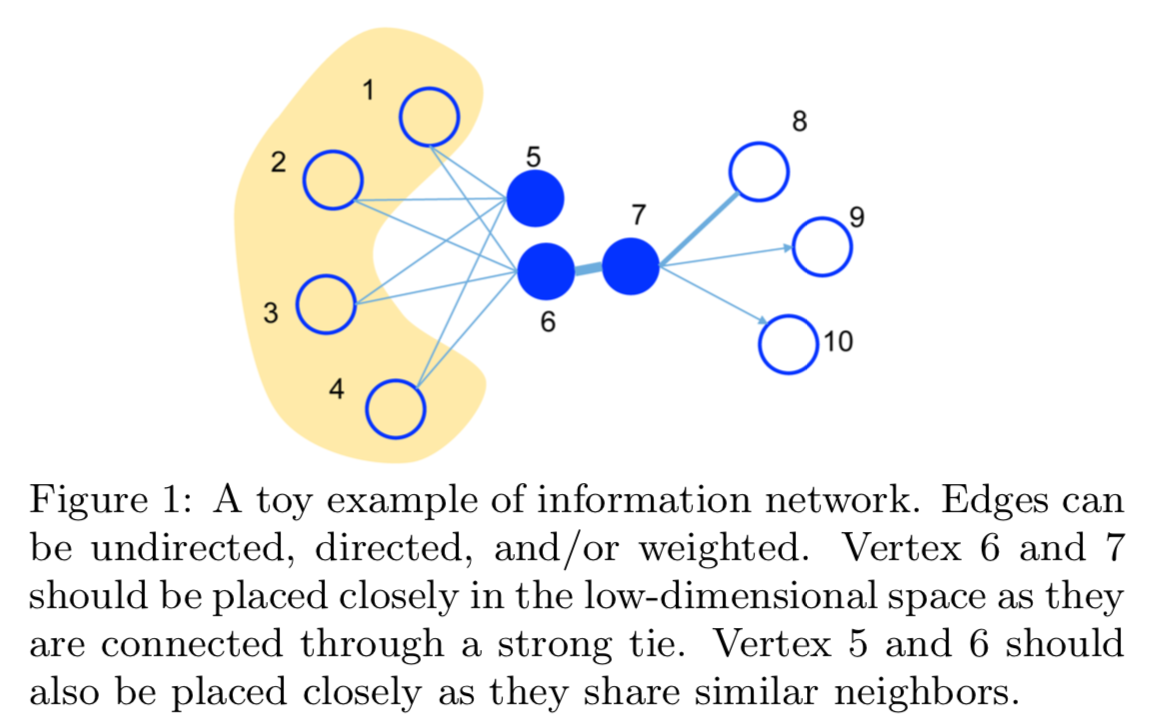### Problem definition

First-order Proximity:

the local pairwise proximity between two vertices

Second-order Proximity:

proximity between a pair of vertices (u,v) in a network is the similarity between their neighborhood network structures

### Large-Scale Information Network Embedding

#### LINE with First-order Proximity

define the joint probability between vertex $v_i$ and $v_j$ as follows:

empirical probability:

objective function:

Replacing d(·, ·) with KL-divergence and omitting some constants, we have:

#### LINE with Second-order Proximity

define the joint probability between vertex $v_i$ and $v_j$ as follows:

empirical probability:

objective function:

Replacing d(·, ·) with KL-divergence and omitting some constants, we have:

### Model Optimization

• negative sampling
• Optimization via Edge Sampling